Plus Two Economics-Chapter 8

# Plus Two Economics-Chapter 8

#### Chapter 8:-

Plus Two Economics Chapter-8 National Income Accounting## Introduction

National income is the income of the nation. Just as a person’s income reflects his economic status, national income reflects the economic status of the nation. All nations aim at improving their national income and per capita income. The performance of an economy can be assessed from the rate of growth of its national income and per capita income. National income figures summarize the performance of the economy. They are absolutely necessary for economic policy formulation and planning. That is why national income accounting is important.

## Basic Concepts

National income is the sum total of the money value of all final goods and services produced in a country during a year. It is the index of economic growth. National income figures are indispensable for economic policy formulation and planning.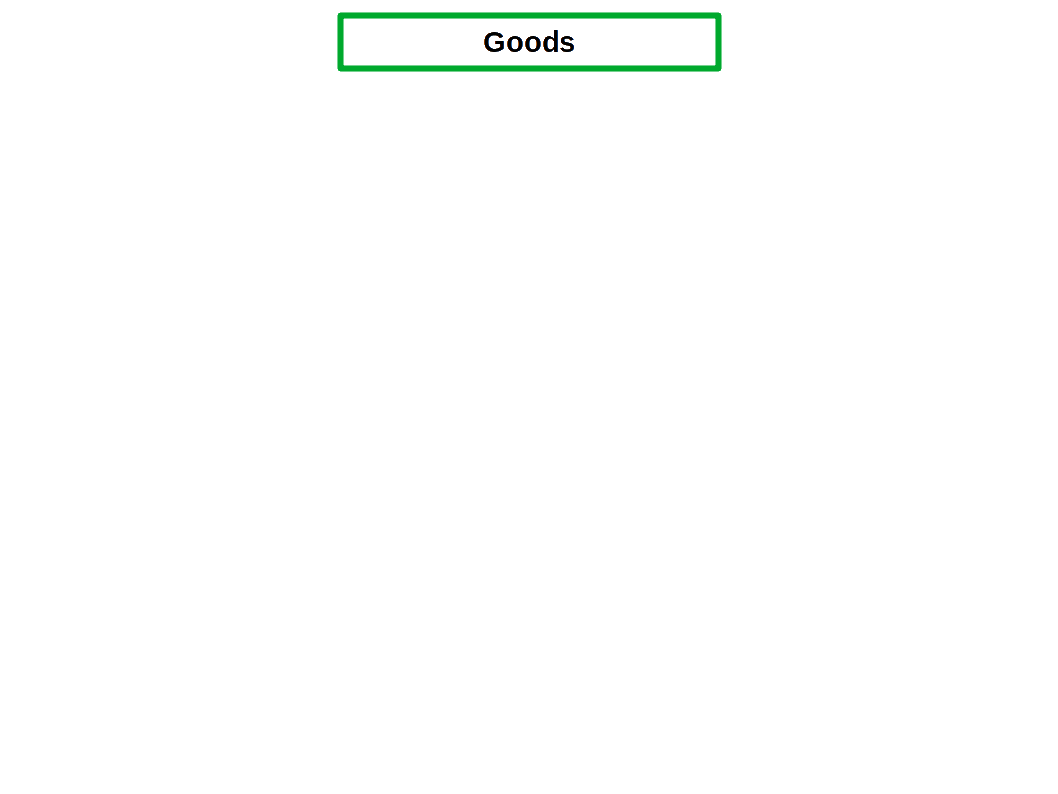## Final goods

Goods used for final consumption and not again subjected to the process of production of other goods are called final goods. For example, clothes, chappals, house, shoes, school bags, cycles, food items, TV, motorcycles, etc.## Intermediate goods

Goods used as an input for producing other goods are called intermediate goods. e.g., Cotton thread used to make cloth, cement, sand, bricks, etc. used to construct houses, leather used to make shoes, plastic material used to make bags, steel used in the manufacture of cycles, wheat used to make bread.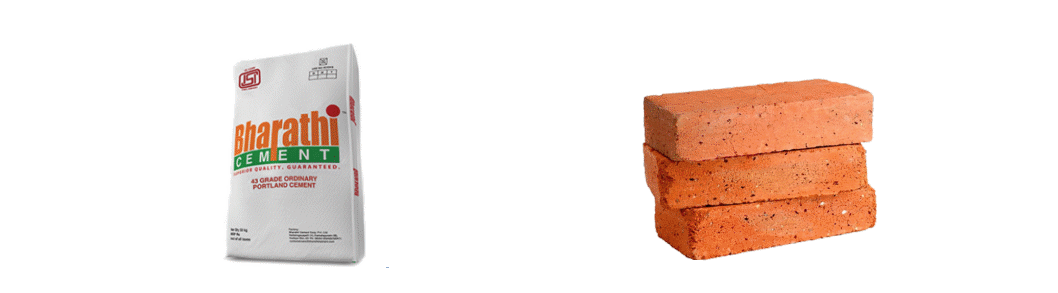## Consumption goods

Goods which are not subjected to a further process of production and used by the consumer directly are called consumption goods or Consumer goods. Consumer goods may be of two kinds:

### Durable goods

Durable goods are those that last long. They can be used again and again. For example, Car, scooter, TV, computer, etc., are durable consumer goods.### Non-durable goods

Goods that last only for a short-time are non-durable goods. Consumer non-durable goods are those goods which perish with consumption. Food products like milk, eggs, vegetables, etc., are non-durable consumer goods.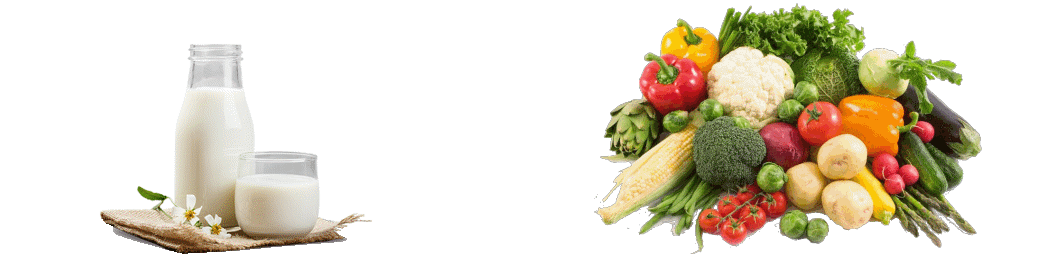## Capital goods

Goods once produced and which can be used again for production are called capital goods. For example, Machinery, factory, buildings, trucks, aeroplanes, roads, ports, etc.## Depreciation or Consumption of Fixed Capital

Wear and tear of capital goods is called depreciation. That is, the loss of value of fixed assets due to normal wear and tear is called depreciation or consumption of fixed capital.

Production units every year set aside a special fund to maintain capital goods in productive condition, This is called depreciation allowance. Capital goods that have suffered wear sand tear should be repaired and replaced. Expenses connected with repair and replacement of capital goods are called depreciation costs.

Suppose a production unit purchases a new machine for ₹10,00,000. After 20 years, this machine has to be replaced due to wear and tear. So every year, the depreciation cost for this machine is ₹ 50,000 (10,00,000 ÷ 20).

## Stocks and Flows

Anything that undergoes changes is called variable. On the basis of measurement, variables are classified into Stocks and Flows.

A variable that can be measured at a point of time is called a stock. A stock is a static concept. It has no time limit. It is measured at a particular point of time. Supply of money, bank deposit, food grain stock, foreign currency reserves, total borrowings, capital, etc., are examples for stock.

A variable that is measured in a specific period of time is called a flow. It is a dynamic concept. It is measured over a period of time. National income, capital formation, foreign remittances to the country, imports, exports, borrowings, etc., are examples of flows.

• Classify the following variables under the heads Stocks and Flows:
• Water in a tank, water that flows through a tap, credit, water in a lake, water in a river, wealth, income, investment, capital formation, supply of money.

Table 8.1
Stocks Flows
Water in a tank Water that flows through a tap
Water in a lake Water in a river
Wealth Income
Investment Capital formation
Supply of money Credit

## Inventory

The quantity of output of a firm that could not be sold is called inventory. Stock of raw materials, semi-finished goods and unsold finished goods which a firm carries from one year to the next is called inventory. Inventory is a stock variable. It may accumulate and decumulate.

If the value of inventory at the end of year is more than the value of inventory at the beginning of the year it is called accumulation of inventory. If the value of inventory at the end of the year is less than that at the beginning of the year it is called decumulation of inventory. So we can find the change in the inventory of a firm during a year by deducting annual sales from production.

Change in inventory (A) = Production during the year (Q) — Sale during the year (V)

At the beginning of a year, a firm had unsold stock worth ₹ 100 of the previous year. During the current year it produced output worth ₹ 1,000, out of which goods worth ₹ 800 was sold. Then difference between production and sale is ₹ 200. (1,000 – 800 = 200). So the change in inventory in the current year is ₹ 200. When this is added to previous year inventory, the current year inventory is ₹ 300.

• A car manufacturing company started production with a stock of 700 cars. During current year the company manufactured 1,200 cars out of which it sold 1,100 cars. Calculate:
1. Change in the current year inventory.
2. Find the inventory at the end of the current year.
• We can find solution to the above question in the below given way.

1. Change in current year inventory = Production — Sale

= 1,200 – 1,100 = 100 cars

2. Inventory at the end of current year = Change in inventory in previous year + Change in inventory in current year

= 700 + 100 = 800 cars.

We can also find the change in inventory by subtracting the closing stock from the opening stock.

Change in inventory = Closing stock — Opening stock

Opening stock is the inventory at the beginning of an accounting year. Closing stock is the inventory at the end of an accounting year.

A firm’s stock as on 1-4-2014 was ₹ 700 crore. Its stock decreased to ₹ 300 crore by 31-3-2015. Therefore, Change in stock = Closing stock – Opening stock = 300 – 700 = ₹ -400 crore. Change in stock can be positive, negative or zero.

### Planned and Unplanned Inventories

Fluctuations in the economy may lead to accumulation and decumulation in inventories of a firm. Increase or decrease in inventory may be planned or unplanned. Deliberate increase in the stock of goods of a firm is called planned accumulation of inventories. Deliberate decrease in the inventories of a firm is called planned decumulation of inventories.

Unexpected increase in the stock of goods due to fall in sales is called unplanned accumulation of inventories. Unexpected decrease in the stock of goods due to rise in‘sales is called unplanned decumulation of inventories. Inventories are considered as a part of capital. Therefore, increase in inventories are considered investment.

## Gross Investment

That part of final output that comprises capital goods is called gross investment of an economy. Roads, railways, airports, bridges, dams, vehicles, machines, tools and instruments, buildings, store houses, etc., are examples of gross investment.

## Net-Investment

In an economy addition to the existing stock of capital is net investment. That is, new capital formation is net investment. Net investment is obtained by deducting depreciation from gross investment.
Net investment = Gross investment — Depreciation

## Net Indirect Taxes

Indirect taxes are taxes imposed by the government on goods and services. Import duty, export duty, excise duty, sales tax, value added tax (VAT), etc., are examples of indirect taxes. Since producers have to remit these taxes to the government, they become part of cost of production, This results in increase in the cost of production. When cost of production increases, price of goods a increases. Thus, when indirect axes increase, price of goods and services also increases.

Sometimes, the government gives subsidies or grants to encourage producers. For example, subsidy is given to handloom products and handicrafts. Subsidies are financial help given to the producers by the government. Subsidies reduce cost of production and thereby reduce prices of goods and services.

As far as producers are concerned, indirect taxes are expenditures, and subsidies are receipts.

Indirect taxes paid by a firm minus the subsidies received by it is called net indirect taxes.

Net indirect taxes = Indirect taxes – Subsidies (NIT = T – S)

## Transfer Payments

Transfer payments are the unilateral payments for which no services are rendered. It is the payment made by an individual, institution or government to another individual, institution or government without getting any service. Taxes, subsidies, scholarships, unemployment pension, old age pension, widow pension, donations, etc., are examples for transfer payments.

Transfer payments are divided into current transfer payments and capital transfer payments. Current Transfer Payments are payments made for current consumption. Taxes and scholarships are examples. Capital Transfer Payments are payments made for capital formation. For example: financial assistance given for construction of house and production units.

## Net Factor Income from Abroad

Net factor income from abroad is the difference between the factor income earned by the domestic factors of production employed in the rest of the world and the factor income earned by factors of production of the rest of the world employed in the domestic economy.

### Macroeconomic Models

Models which are framed for simplifying complex economic issues based on certain assumptions are called Macroeconomic models. These are extensively used for the formulation of policies and programmes, and their implementation. These models do not depict an actual economy in detail.

## Circular Flow of Income

Circular flow of income and expenditure -is the pictorial illustration of the interrelationship and interdependence among different sectors of the economy. There are different models of circular flow of income. A very simple economic model among them is the two-sector model.

### Circular Flow of Income in a Simple Economy

Circular flow of income in a simple economy is the circular flow of income of two sectors in the economy. This simple two-sector model is based on the following assumptions:

1. There are only two sectors in the economy — households and firms.
2. Households supply factor services. Firms buy the factors of production.
3. Households spend their entire income on consumption. Nothing is saved.
4. Firms sell their entire output to the households.
5. The economy is a closed economy without government.

This simple two sector model has two markets. (1) Product Market for goods and services and (2) Factor Market for factors of production. The two sector model of circular flow is given in the below Diagram.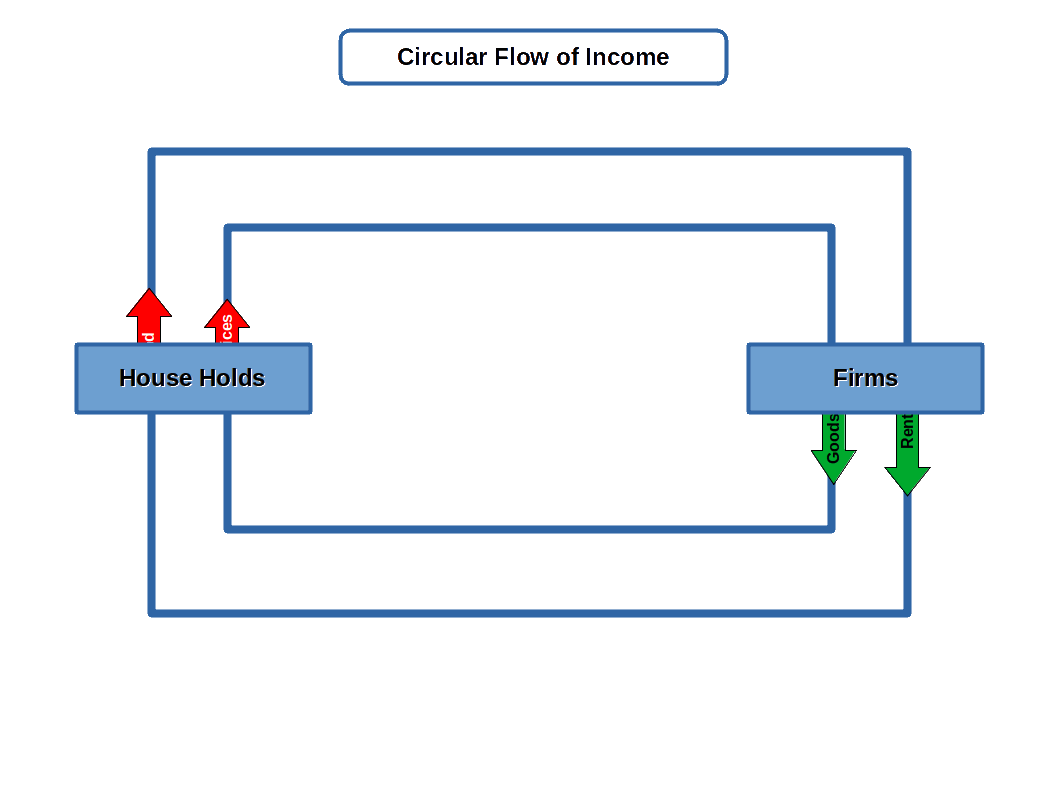The main activity of the households is supply of factors of production. The household sector gives factors of productions they own, namely land, labour, capital and entrepreneurship to firms. This is the first flow. Using these factors of production, the firms produce goods and services. They reward the owners of the factors of production in the form of rent, wages, interest and profit. This is the second flow.

Reward given by the production units is called factor payments. Rewards received by the owners of the factors of production is called factor income. Factor payment and factor income are one and the same.

Households use the factor income to buy goods and services made by the firm, In this way money flows from households to firms. The money that the households give to the firms to buy goods and services is the third flow. In return, the firms give goods and services to the households. This is the fourth flow. Thus circular flow is complete. In short, flow of factors of production, flow of factor payment or factor income, flow of expenditure and flow of goods and services between households and firms is called the circular flow of income.

There are two types of flows:

1. Money flow and
2. real flow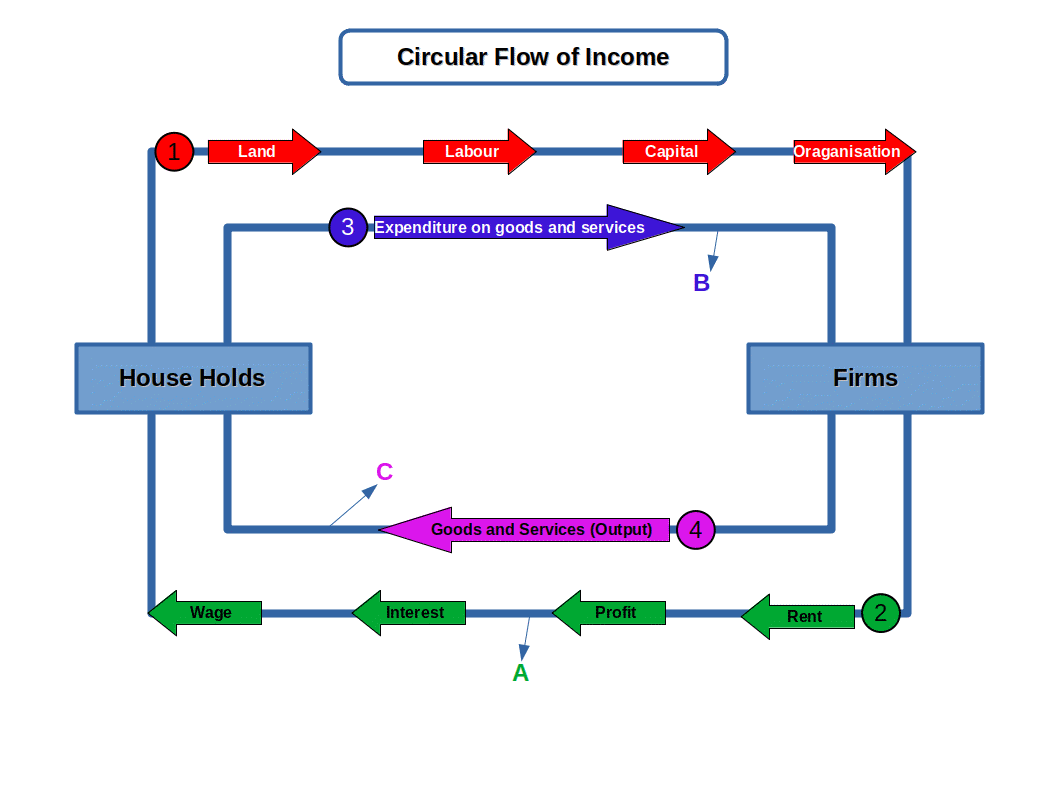The flow of money from firms to households and from households to firms is called Money flow (second and third flow). The flow in the form of goods and services from one sector to another is called Real flow (first and fourth flow).

Table 8.2
Real flows Money flows
Factors of production (land, labour, capital and organisation) Factor payments (rent, wages, interest and profit)
Goods and services Price of goods and services

We can calculate the annual total income or national income of an economy at the points A, B and C in the above Diagram. If the money value of output is measured as a flow of income at point A it is called income method. If the money value of output is measured as a flow of expenditure at point B it is called expenditure method. If money value of output is measured as a flow of goods at point C it is called the product method.

## Measurement of National Income

There are three methods of measurement of national income. They are:

3. ### Income method

These methods of calculations of GDP are discussed in detail.

### 1. Product Method or Value added Method

Under this method the aggregate of the value of goods and services produced is calculated by adding all the final goods and services produced by each production unit in the economy in a given period of time usually, a year. Here calculation is made from the output side. The final goods and services produced by the production units can be called output. The value of output is determined on the basis of the prevailing market price of output. The value of output or money value of output is obtained by multiplying the quantity of output by prevailing market price. That is,

Value of output (Q) = Market price(P) × Output (q)

It is very difficult to determine the exact value of the final output, because the final output produced by a firm is used as input in other firms. In this situation there is a possibility that the value of output of a good is added more than once.

When output of a good or service is added more than once in calculation of national income it is called double counting.

To avoid double counting, value added method is used to calculate final value of output of a firm. Value added or Gross value added is the net contribution made by a firm at different stages of production. It is obtained by subtracting value of intermediate consumption from Gross value of output.
Gross Value Added (GVA) = Gross value of output (Q) – Value of intermediate consumption (Z)

Use of intermediate goods of a firm purchased from other firms is called intermediate consumption. For example, a bread making company uses wheat produced by farmers, and a car making company uses tyres produced by another company.

For example, a farmer produces wheat worth ₹ 100 by using his labour as the only input and sells to a baker who produces bread. The baker makes bread and sells it to consumers for ₹ 250. The value of output and value added of the farmer are equal, because the intermediate consumption of the farmer is assumed to be nil. This is ₹ 100. The value added by the baker is ₹ 150 (250 – 100 = 150). This is given in the following table:

Table 8.3
Producers Name of Product Gross value of output (Q) Intermediate Consumption (Z) Value Added (Q – Z)
Frmer Wheat 100 Nil 100
Total 350 100 250

If we add the final value Of output of every producer we get 100 + 250 = ₹ 350. The value of wheat ₹ 100 is included in the value of bread. So the value of wheat is added twice. First, as the output of the farmer and second, as the output of the baker for making bread. This is double counting. Really, total gross value of output is ₹ 250 of which net contribution of farmer is ₹ 100 and net contribution of baker is ₹ 150.

When production takes place, fixed capital undergoes wear and tear (depreciation) due to the process of production. If depreciation charge is included in the value added it is called Gross Value Added (GVA). If value of depreciation is deducted from Gross value added, it is called Net Value Added (NVA).

Net value added = Gross value added – Consumption of fixed capital / depreciation

For example, when a firm produces goods worth ₹ 100, the value of intermediate consumption is ₹ 20 and depreciation is ₹ 10. Then, gross value added = 100 – 20 = ₹ 80. Net value added = 80 – 10 = ₹ 70.

Notes:

• The symbol Σ (sigma) denotes summation
• $$\sum_{i=0}^{n} X_i$$ is equal to X1 + X2 + … + Xn
• The sign ‘≡’ stands for identity.

A firm’s Gross Value Added during a year can be calculated in two ways:

• Gross value added of firm i (GVAi) = Gross value of the output produced by the firm i(Qi) – Value of intermediate goods used by firm i (Zi) and
• GVAi = Value of sales by the firm i(Vi) + Value of change in inventories of firm i (Ai) – Value of intermediate goods used by the firm (Zi).

Gross value added of firm i (GVAi) = Gross value of the output produced by the firm i(Qi) – Value of intermediate goods used by firm i (Zi) and GVAi = Value of sales by the firm i(Vi) + Value of change in inventories of firm i (Ai) – Value of intermediate goods used by the firm (Zi).

2. ### GVAi = Vi + Ai – Zi

As per Value Added Method, GDP is the sum of Gross Values Added by all the producers in the domestic territory of an economy. during a year. If there are N production units in the domestic economy of a country, GDP is the sum of gross values added by N firms. Mathematically,

GDP ≡ GVA1 + GVA2 + … + GVAN ≡ $$\sum_{i=1}^{N} GVA_i$$

NVAi is the Net value added of the firm i and Di is the depreciation of firm i. Then, NVAi ≡ GVAi – Di and GVAi ≡ NVAi + Di.

If there are N number of firms, then

$$GDP\, ≡\, \sum_{i=1}^{N} \,NVA_i\,+\,\sum_{i=1}^{N}D_i$$

This indicates that GDP is the sum total of net value added and depreciation by all the firms in an economy.

NDP (Net Domestic Product) is the sum vf the Net Values Added by all firms.

$$NDP\, ≡\, \sum_{i=1}^{N} \,NVA_i\$$

When we add Net Factor Income from Abroad (NFIA) with Net Domestic Product at Factor Cost (NDPfc), we get National Income (NNPfc).

### 2. Expenditure method

The method of calculating National Income on the basis of the final expenditure on domestic product is called expenditure method or outlay method. Under this method, GDP is calculated by adding the final expenditures in the domestic territory of a country. This is the expenditures of households, firms, government and the rest of the world incurred to purchase final goods produced by all the firms in the domestic territory. Expenditure on intermediate goods will not be considered.

Firm i makes the final expenditure into four accounts:

1. Final consumption expenditure on goods and services produced by the firm i from households (C).
2. Final investment expenditure incurred by other firms on capital goods produced by firm i (I).
3. Government final consumption expenditure on goods and services produced by firm i (G).
4. Export revenues that firm i earns by selling its goods and services abroad (Xi).
All the expenditures of the firm i earned through final consumption, investment, government consumption and exports are equal to the total revenue of the firm i. So revenue value of firm i is

$$RV_{i}\, ≡\, C_{i}\,+\,I_{i}\,+\,G_{i}\,+\,X_{i}$$

Thus, if there are N firms in the economy, total revenue earned by these firms is the sum of expenditures of final consumption, investment, government consumption and exports. That is,Aggregate expenditure of household sector, firms and governments of an economy is the expenditure on goods and services produced by (1) domestic firms and (2) foreign firms. Expenditure on the firms in the rest of the world is called import expenditure. This is not the expenditure on goods produced in the domestic economy. Therefore, to find the expenditure on the GDP produced by domestic firms, deduct import expenditure from total expenditure. The aggregate final consumption expenditure of the economy (C) is the sum of final consumption expenditure on goods and services produced in the domestic economy $$\Bigl(\sum_{i=1}^{N}C_{i} \Bigr)$$and final consumption expenditure on goods and services imported (C m). Therefore aggregate final consumption expenditure is,

$$C_{i}\, ≡\, \sum_{i=1}^{N} \,C_{i}\,+\,C_{m}$$ $$∴\, \sum_{i=1}^{N} \,C_{i}\,≡\,C\,-\,C_{m}\,\,(2)$$

C – Cm is the final consumption expenditure on domestic goods and services.

Similarly, aggregate final investment expenditure of the economy (I) is the sum of final investment expenditure on domestic firms $$\Bigl(\sum_{i=1}^{N}I_{i} \Bigr)$$ and the final investment expenditure on imported investment goods (Im ). So aggregate final investment expenditure is,

$$I_{i}\, ≡\, \sum_{i=1}^{N} \,I_{i}\,+\,I_{m}$$ $$∴\, \sum_{i=1}^{N} \,I_{i}\,≡\,I\,-\,I_{m}\,\,(3)$$

I – Im is the final investment expenditure on capital goods produced in the domestic territory. The aggregate government expenditure of the country (G) is the sum of final government expenditure on domestic firms $$\Bigl(\sum_{i=1}^{N}G_{i} \Bigr)$$ and final government expenditure on foreign goods and services (G). This is the import expenditure of the Government. Therefore Government aggregate expenditure is,

$$G_{i}\, ≡\, \sum_{i=1}^{N} \,G_{i}\,+\,G_{m}$$

$$∴\, \sum_{i=1}^{N} \,G_{i}\,≡\,G\,-\,G_{m}\,\,(4)$$

G – Gm is the Government expenditure on domestic firms. Under expenditure method, we know that GDP is the aggregate final expenditure received by all the firms in the domestic economy. Symbolically,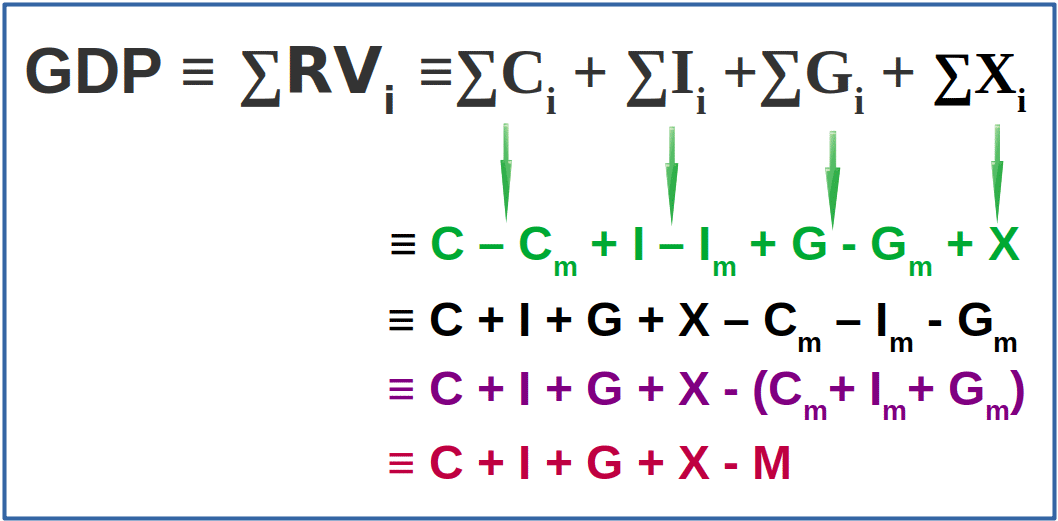M = Cm + Im + Gm is the total import expenditure of the economy.This is the GDP as per expenditure method.

### 3. Income Method

Under income method GDP is calculated by adding together all the factor income received by the owners of factors of production, such as land, labour, capital and entrepreneurship in the domestic economy in the form of wages, rent, interest and gross profit. Here depreciation charges and net indirect taxes are included in gross profit.

Let Wi, Ri, Ini, and Pi be the wages & salaries, rent, interest and gross profit respectively of i-th household in the economy in a year. Wi + Ri + Ini + Pi is the annual income received by i-th household. If there are N house holds in the domestic territory the total income of N households will be equal to GDP. i.e.,$${GDP\,≡\,W+R+In+P}$$

whereTherefore, as per income method, the GDP of an economy is the sum total of wages, rent, interest and gross profit received by all the factors of production.

For example, Wages = ₹ 750 crore, Rent = ₹ 350 crore, Interest = ₹ 200 crore and Gross profit = ₹ 300 crore are the factor rewards received by household for supplying factor services in the economy in a year. Therefore,

$${GDP\,≡\,W+R+In+P}$$

= 750 +350 + 200 + 300

= ₹1,600 crore

## Reconciliation of the three methods of measuring National Income

The three methods approach the computation of national income in three different ways. Though the methods are different, the value of national income would be same. The value of GDP derived from these three methods must be identical.• From the table, find GDP using. Product Method, Expenditure Method and Income Method.

• Depreciation (D) Import (M) Table 8.4 Items Amount 20 50 100 150 200 200 250 300 400 500 1300

GDP by Product Method

$$GDP\, ≡\, \sum_{i=1}^{N} \,Q_{i}\,-\,\sum_{i=1}^{N} \,Z_{i}$$

$$1300 \,-\,400\,=\,₹\,900$$

GDP by Expenditure Method

$${GDP\,≡\,C+I+G+X-M}$$ $${=\,500+200+150+100-50}$$ $$=\,₹\,900$$ GDP by Income Method

$${GDP\,≡\,W+R+In+P}$$ $${=\,300+150+200+250}$$ $$=\,₹\,900$$

## Budget Deficit and Trade Deficit

Household sector uses a part of their income for consumption (C), another part for paying tax (T) and the remaining part for saving (S).

Therefore,

$$GDP ≡ C+S+T$$

As per Expenditure method, GDP ≡ C + I + G + X – M

C + I + G + X – M ≡ C + S + T

By cancelling C on both sides,

I + G + X – M ≡ S + T

By rearranging,

I – S + G – T ≡ M – X

G – T is the difference between Government income and expenditure. When Government expenditure is more than the income it is called Budget Deficit.

M – X stands for the difference between import expenditure and export income. If import expenditure is more than export revenue it is called Trade Deficit.

In a closed economy where there is no Government and foreign trade,

$$\mathbf{I\,≡\,S}$$

Rent, interest and profit are called operating surplus. Profit is the sum of dividend, undistributed profit and corporation tax.

## Some Macroeconomic Identities

National income and other macroeconomic aggregates are used to interpret economic performance. For example, GDP is the index of economic growth. Rate of economic growth is the rate of growth of GDP at constant prices. Similarly, per capita income is the index of standard of living of the people. All these concepts are derived from national income, and they are related to each other. Now we shall study these concepts.

## National Income (NI)

National income is the income of the people of a nation in a year. It includes the income of people working abroad. We can arrive at National income by deducting depreciation and net indirect taxes from GDP, and adding net factor income from abroad. The aggregate thus obtained is known as Net National Product at factor cost (NNPFC).

NI ≡ NNPFC ≡ GDPMP – Depreciation – Net Indirect Taxes + Net Factor Income from abroad

National income and macro aggregates are expressed either in market prices or factor cost. We can understand these concepts from the below given Diagram.### (1) Gross Domestic Product (GDP)

Gross domestic product is the total money value of all final goods and services produced in the domestic territory of a country in a year.### (2) Gross National Product (GNP)

Gross National Product is defined as the sum of GDP and net factor income from abroad (NFI).### (3)Net National Product (NNP)

Net National Product is the total money value of all final goods and services produced by a country in a year less depreciation.### (4) Net Domestic Product (NDP)

Net Domestic Product is the total money value of all final goods and services produced in the domestic territory of a country less depreciation.NDPFC can be called Domestic Factor Income.

###1. Difference between National product and Domestic product is NFI
2. Difference between Gross product and Net product is Depreciation
3. Difference between market price and factor price is NIT

• W = 800, R = 300, In = 400, P = 200, D = 5O, NIT = 100 and NFI = 150 ( in crore) are the components of GNP of a country
• GNPMP = W + R + In +P + D + NIT + NFI

= 800 + 300 + 400 + 200 + 50 + 100 + 150 = ₹ 2,000 crore

GNPFC = GNPMP – NIT

= 2,000 -100 = ₹ 1,900 crore

NNPMP = GNPMP – D

= 2,000 – 50 = ₹ 1,950 crore

NNPFC = NI = NNPMP – NIT

= 1,950 – 100 = ₹ 1,850 crore

GDPMP = GNPMP – NFI

= 2,000 – 150 = ₹ 1,850 crore

GDPFC = GDPMP – NIT

= 1,850 – 100 = ₹ 1,750 crore

NDPMP =_GDPMP – D

= 1,850 – 50 = ₹ 1,800 crore

NDPFC = DFI = NDPMP – NIT

= 1,800 – 100 = ₹ 1,700 crore

### (5) National Disposable Income – NDI

National disposable income is the income from all sources available to the residents of a country for consumption expenditure and saving for one year.

NDI ≡ NNPMP + current transfers from the rest of the world

### (6) Personal Income

Personal Income is that part of national income which is received by households. It is the income available to persons from all legal sources.

PI ≡ NI – Undistributed profits – Net interest payments of households – corporate tax + transfer payments.

### (7) Personal Disposable Income – PDI

Income which individuals, and households can spend as they wish is called Personal Disposable Income.

PDI ≡ PI – (Personal tax payments + Personal non-tax payments)

### (8) Per Capita Income – PCI

Per capita income is the annual average per head income of the people of a country. It is calculated by dividing national income by population.

$$PCI = {\frac {National\,Income} { Population}}$$

### (9) Private Income

Net factor income from the employees and private entrepreneurs in the domestic sector, net factor income from abroad, net transfer of payments of Government and the rest of the world and interest on national borrowings constitute private income.

Private Income = factor income from net domestic product accruing to the private sector + national debt interest + net factor income from abroad + current transfers from government + other net transfers from the rest of the world.

## Goods and Prices

GDP is affected by changes in price level. An increase in the price level reflects an increase in GDP and a decrease in price level reflects a decrease in GDP. To eliminate the effect of change in price level on GDP economists use nominal GDP and Real GDP.

## Nominal GDP

The Gross domestic product in which the money value of goods and services calculated on the basis of current price is called nominal GDP.

In order to calculate nominal GDP, prices prevailing in the year in which the GDP under consideration is taken into account. That is, GDP at current prices is called nominal GDP. Nominal GDP is denoted by GDP.

Current prices are the prices prevailing in the year of production.

## Real GDP (gdp)

Gross domestic product in which the money value of goods and services calculated on the basis of constant prices is called real GDP and is denoted by gdp. Here, the value of goods and services is determined on the basis of base year prices or constant prices.

## GDP Deflator

The GDP deflator gives us an idea of how the prices have moved from the base year to the current year. It is a good index for measuring price level. GDP deflator is the ratio of nominal GDP to real GDP. It is calculated by dividing nominal GDP by real GDP. It is also expressed as percentages.

$$GDP\,Deflator\,= {\frac {GDP} {gdp}}$$

$$or$$

$${\frac {GDP} {gdp}}\,×\,100$$

Nominal GDP for the year 2001-02 is ₹ 1,650 and real GDP is ₹ 1,100. The GDP deflator is 1.50 (1,650 ÷ 1,100 = 1.50). In percentages, it is 150. (1,650 ÷ 1,100 × 100 = 150).

• GDP of a country at current price is ₹ 2,000 crore and GDP at constant price is ₹ 3,000 crore. Find the GDP deflator and interpret.
• $$GDP\,Deflator\,= {\frac {GDP} {gdp}} \,×\,100$$

$$=\, {\frac {2000} {3000}} \,×\,100$$ $$=\,66.66$$

This implies that the general price level in the country has decreased compared to base year Prices. i.e, prices in the base year ₹ 100 came down to ₹ 66.66 in the current year.

## Consumer Price Index – CPI

Consumer Price Index is the index of prices of a given basket of commodities bought by a representative consumer. CPI is the ratio of the cost of purchase of a given basket of commodities in the current year to the cost of purchase of the same basket in the base year. It is generally expressed in percentage terms.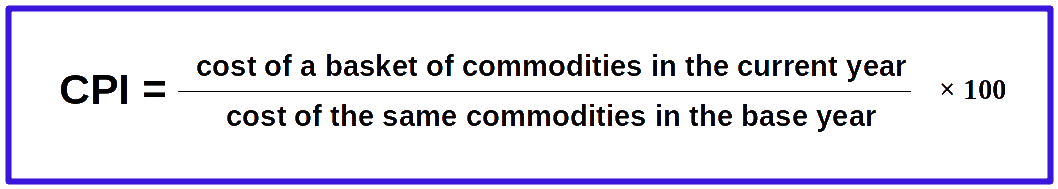## Wholesale Price Index — WPI

Wholesale price index is calculated on the basis of the price existing in the wholesale market where goods or semi-finished goods or raw materials are traded in bulk. They are generally purchased by traders. Therefore, the WPI is calculated on the wholesale price of a given basket of goods which are traded in bulk. WPI is the index of change in price of a basket of goods traded between two different periods of time. In countries like USA, WPI is known as Producers’ Price Index (PPI).

Only goods usually bought by consumers are included All goods and services in the economy are included Table 8.5 Comparison of CPI and WPI CPI WPI

## GDP and Welfare

The ultimate objective of economic policy is to promote the welfare of the people. In reality, can we treat GDP as the index of welfare?

But GDP and its growth rate alone cannot be considered an indicator of development. There can be situations where GDP growth does not lead to promotion of welfare. The following 4 points will justify these arguments.

## 1. Inequality in the distribution of incomeAlong with increase in GDP inequalities in income may occur. Even if the majority does not experience any increase in income, the GDP will increase if the income of a minority increases. It is even possible that a minority may become richer while the majority becomes poorer. Here welfare diminishes even when GDP increases. Therefore, in this context GDP is not a good indicator of welfare.

## 2. GDP and non-monetary exchangesMany of the non-monetary exchanges are not included in the GDP. For example, services rendered by housewives are not included in GDP. Similarly, exchange of goods (barter transactions) also does not come under GDP. To this extent, GDP is inadequate. In a country like India, with huge population and non- monetised sectors, GDP is underestimated. Therefore, considering GDP as an indicator of welfare is subject to much criticism.

## 3. GDP and harmful goodsWhile calculating GDP all goods and services are considered. Whether these goods and services are useful or harmful to the people is not taken into consideration. For example, production of cigarettes and alcohol, both injurious to the health of the people, is included in GDP. So, if production of tobacco and alcohol is increased, GDP will go up. But we cannot say that increase in GDP indicates an improvement in welfare of the people.

## 4. GDP and externalitiesExternalities are unintended consequences of an action. Externalities may be beneficial or harmful. If a good road is constructed through an area which formerly had no good road, the value of land on either side of the road goes up. This is beneficial externality. Similarly, if an IT Park comes up in an area, there will be lots of beneficial externalities. Externalities can be harmful also. If a chemical factory is set up in an area and if it pollutes the nearby river by discharging its effluents, there will be many harmful consequences. People who bathe in the river may get skin diseases; fishermen may lose their livelihood. While calculating GDP, these welfare enhancing and welfare deduction externalities are not considered.